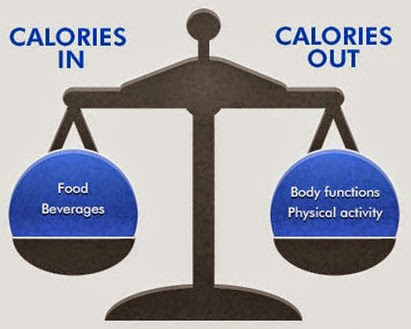# Calculation of Caloric requirements of a normal and sick personWhen you become ill or after an acute injury, you need more energy and calories to recover. You should take the right amount of food in order to make sure that you are getting enough calories.
Now a question arises, how do you know about your caloric requirement? In order to do that, we have a formula and a chart.
The formula to calculate calorie requirement is as follows;

Total Calorie Requirement = (Basal Energy Expenditure) x (Activity Factor) x (Injury Factor)

Now we have to put 3 values in this formula to get the result in the form of total calorie requirement.

### Basal Energy Expenditure

First of all, let us find out the way of calculating basal energy expenditure. Basal energy expenditure is 25/kg for a normal person. So in order to calculate your basal energy expenditure use the following formula.
Basal Energy Expenditure = (25) x (Weight in Kg)
So by using the above formula, we will get the basal energy expenditure.

### Activity Factor

Next in the formula is an activity factor.
If you are confined to bed then your activity factor is 1.2. If you are not confined to bed and are able to get out of bed then your activity factor is 1.3.

### Injury Factor

Next in the formula is the injury factor.
Injury factor varies with the extent and nature of the injury.
If there’s no injury and you are perfectly normal then your injury factor will be 1.
If you have some problem then use an injury factor according to the details given below.

INFECTION

TRAUMA

SURGERY

BURNS
##### Severity of Injury

MILD
MODERATE
SEVERE

SKELETAL
BLUNT

MINOR
MAJOR

0-40%
41-100%

###### Injury Factor

1.2
1.5
1.8

1.35
1.35
1.6

1.1
1.2

1.50
2.1
Now you have all the three variables to calculate your or your patient’s total calorie requirement.
Let’s us take an example.
Let’s say your weight is 70 kg and you’re suffering from mild sore throat and otherwise you’re healthy and aren’t confined to bed.
So your basal energy expenditure can be calculated by using this formula, (also see the formula of BEE above)
Basal energy expenditure = 25 x 70 = 1750 calories
Your activity factor = 1.3 (as you aren’t confined to bed)
and your injury factor = 1.2 (as you’re suffering from mild sore throat).
Now we have the values of all the variables that are need to calculate total calorie requirement.
Now, as we know that;
Total calorie requirement (TCR) = (basal energy expenditure) x (activity factor) x (injury factor)
TCR = 1750 x 1.3 x 1.2
So Total Calorie requirement = 2730 calories.
So you need 2730 calories daily in order to recover quickly and maintain your current status of weight and health.

Also, See;